# In the figure, a bar of length L is attached by a frictionless hinge to a...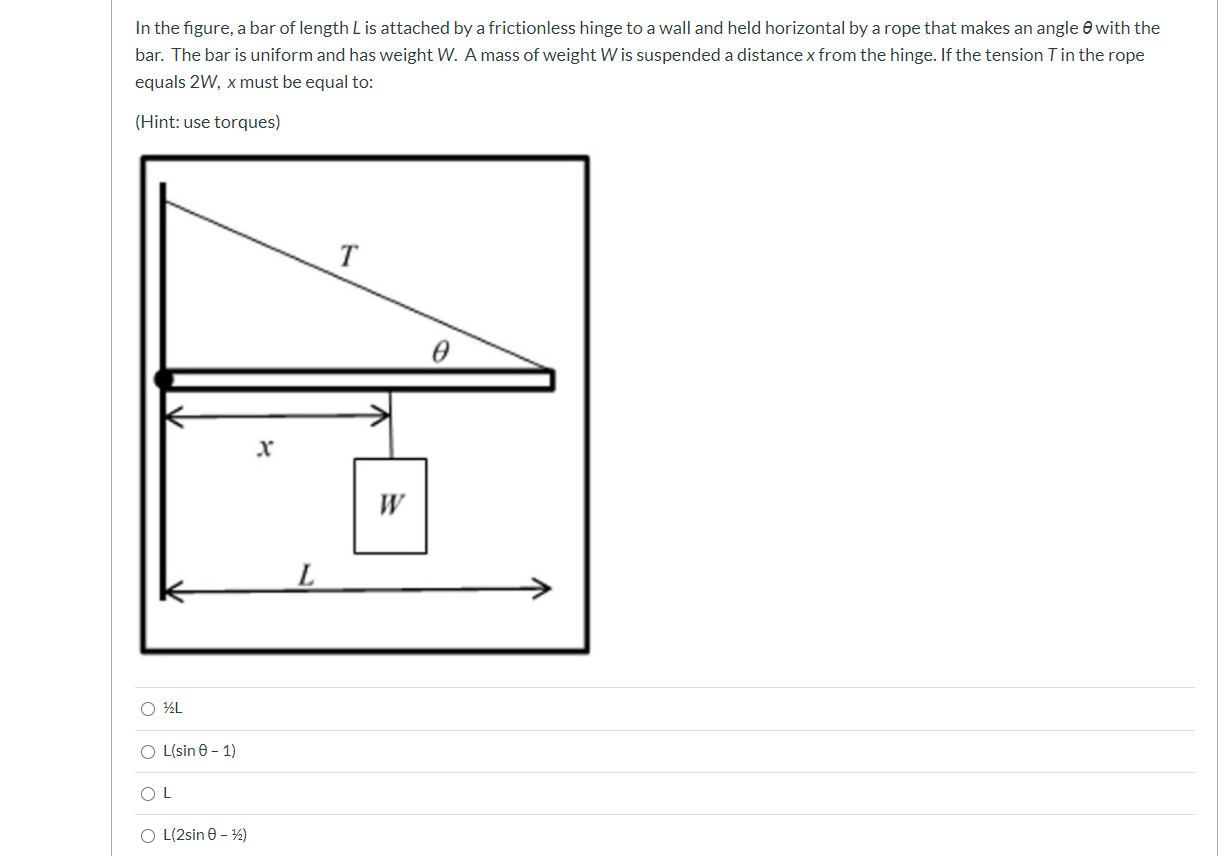In the figure, a bar of length L is attached by a frictionless hinge to a wall and held horizontal by a rope that makes an angle with the bar. The bar is uniform and has weight W. A mass of weight Wis suspended a distance x from the hinge. If the tension T in the rope equals 2W, x must be equal to: (Hint: use torques) T L O OL(sin 0-1) OL OL(2sin - 2)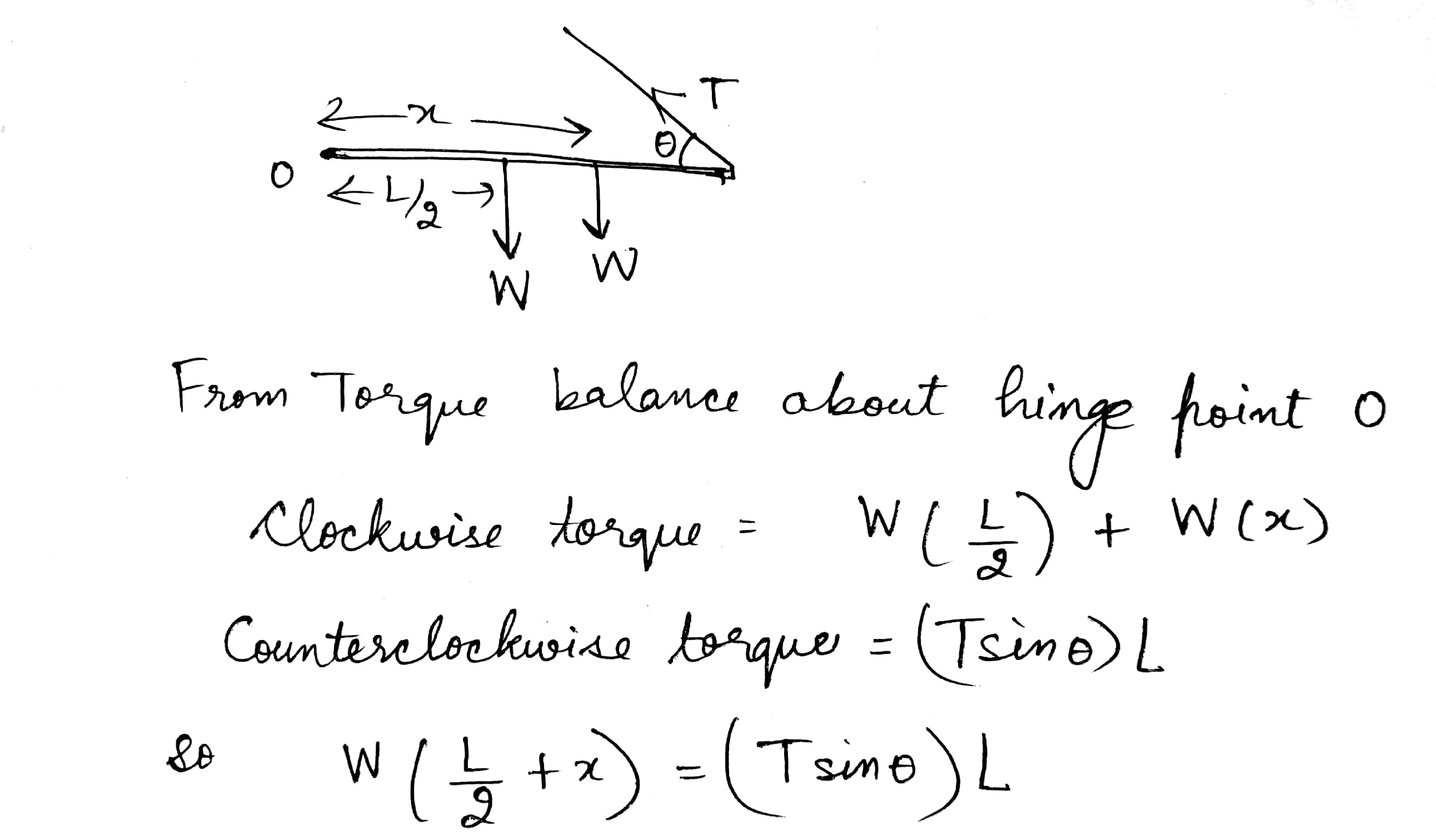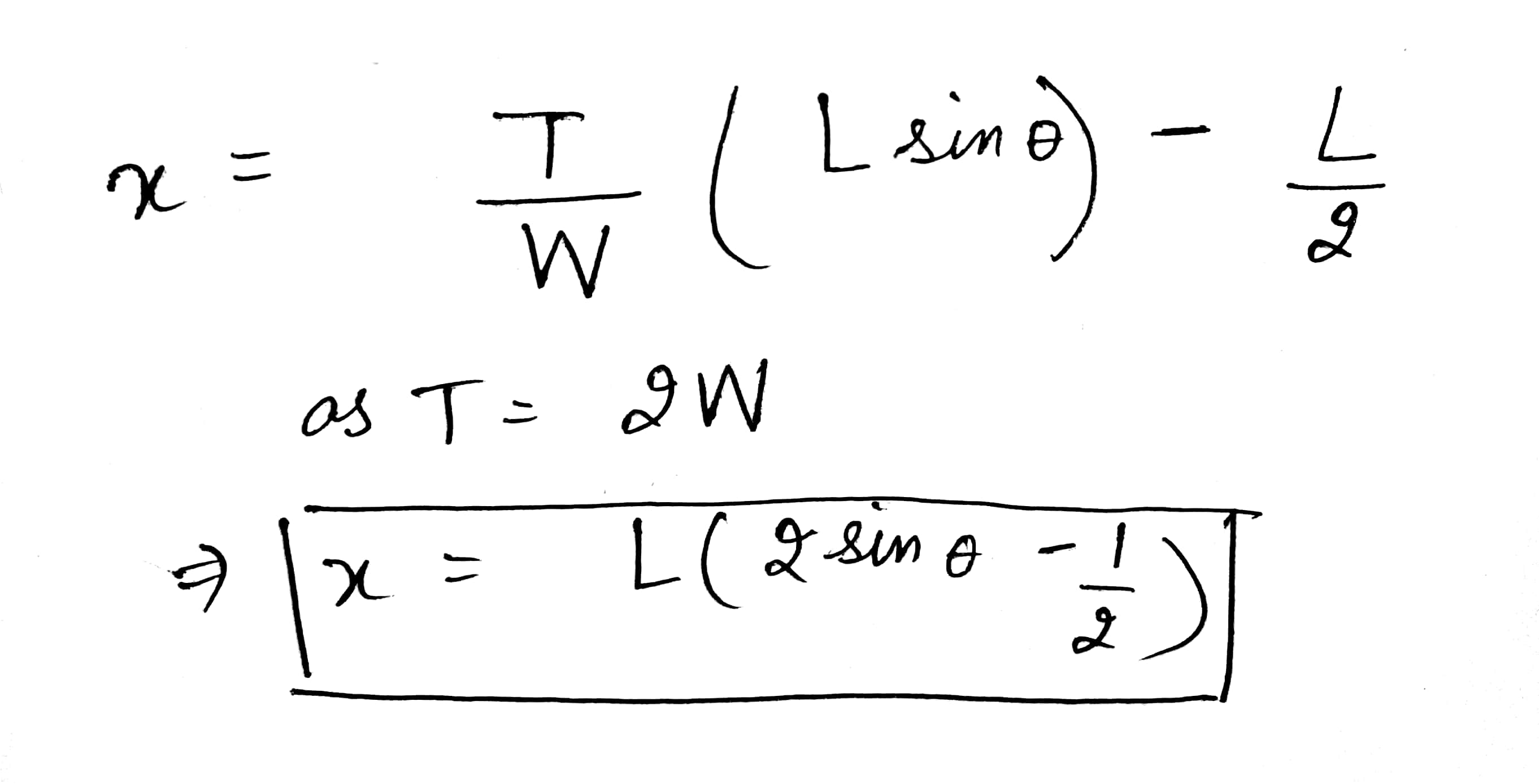please rate it up thanks :)

##### Add Answer of: In the figure, a bar of length L is attached by a frictionless hinge to a...
Similar Homework Help Questions
• ### please show all work 16) In the figure, a 10.0-m long bar is attached by a frictionless hinge to a wall and held hor...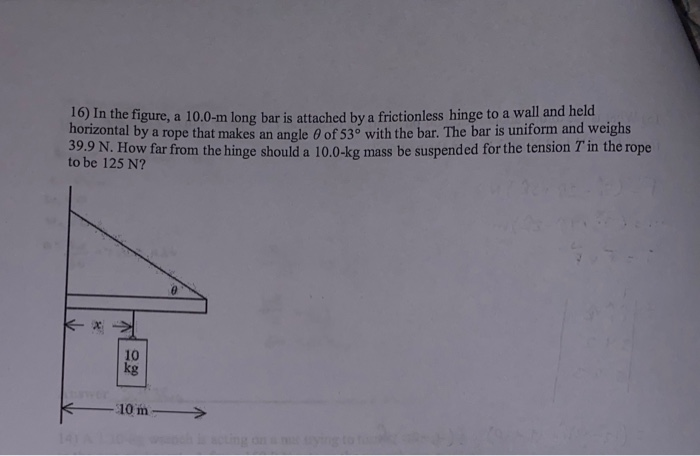please show all work 16) In the figure, a 10.0-m long bar is attached by a frictionless hinge to a wall and held horizontal by a rope that makes an angle 0 of 53° with the bar. The bar is uniform and weighs 39.9 N. How far from the hinge should a 10.0-kg mass be suspend ed for the tension T in the rope to be 125 N? 10 kg 10 m ctin

• ### A uniform bar 7.5 m long is held against a rough wall by a light rope...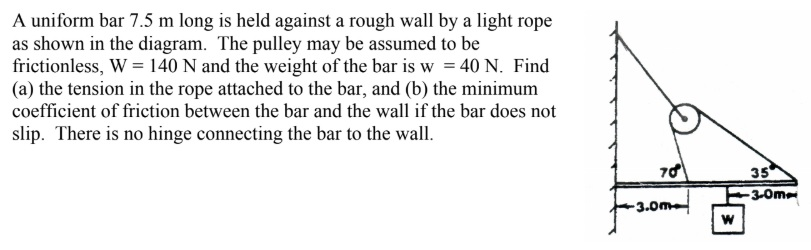A uniform bar 7.5 m long is held against a rough wall by a light rope as shown in the diagram. The pulley may be assumed to be frictionless, W = 140 N and the weight of the bar is w = 40 N. Find (a) the tension in the rope attached to the bar, and (b) the minimum coefficient of friction between the bar and the wall if the bar does not slip. There is no hinge connecting the...

• ### a) Draw fully labelled free body and extended free body diagrams for the object. b) Explain what causes the object t...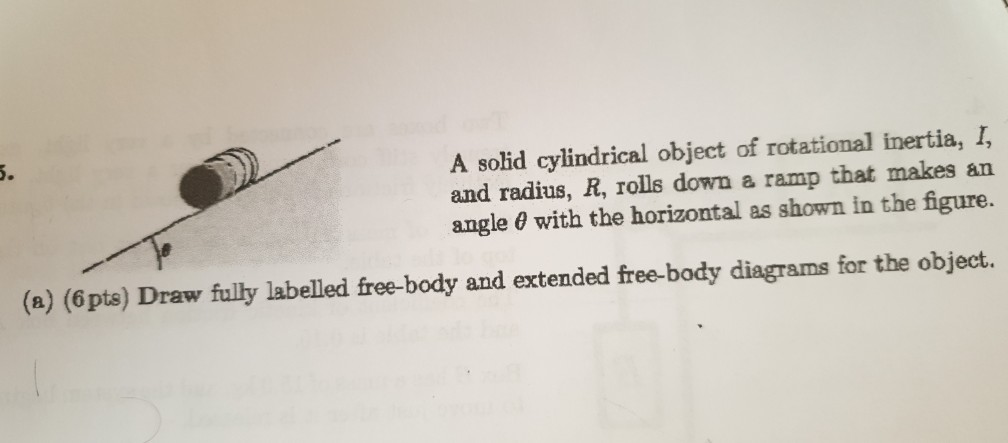a) Draw fully labelled free body and extended free body diagrams for the object. b) Explain what causes the object to roll instead of slide. 2. Estimate how far the Earth moves when you jump up to a height of 10 cm. Hint: The inertia of the earth is about 10^25 kg and its radius is about 10^5 km. 3. 5. A solid cylindrical object of rotational inertia, I, and radius, R, rolls down a angle e with the horizontal...

• ### finding magnitude of force

A uniform bar with mass 50kg and length 4.0m is attached to a wall by a frictionless hinge. The bar is held in a horizontal position by a light rope that is attachedat the the end of the bar. The other end of the rope is attached to the wall. The rope makes an angle of 30 degrees with the wall.What is the magnitude of the resultant force that the hinge exerts on the bar?

• ### A uniform meter stick of mass 1.5kg is attached to the wall by a frictionless hinge at one end. On the opposite end it i...

A uniform meter stick of mass 1.5kg is attached to the wall by a frictionless hinge at one end. On the opposite end it is supported by a vertical massless string such that the stick makes an angle of 40 degrees with the horizontal. Find the tension in the string and the magnitude and direction of the force exerted on the stick by the hinge.

• ### In the figure, a thin horizontal bar AB of negligible weight and length L is hinged...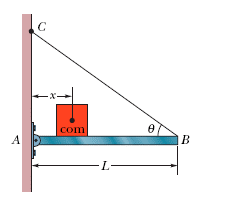In the figure, a thin horizontal bar AB of negligible weight and length L is hinged to a vertical wall at A and supported at B by a thin wire BC that makes an angle θ with the horizontal. A block of weight W can be moved anywhere along the bar; its position is defined by the distance x from the wall to its center of mass. Find vertical components of the force on the bar from the hinge at...

• ### Practice Exam Question

<p>One end of a uniform bar that has a weight of 60N is attached to a wall by a frictionless hinge. The bar held at 53 degrees above the horizontal &#160;bya horizontal cable. What is the tension in the cable?</p>

• ### In the figure, suppose the length L of the uniform bar is 2.8 m and its...

In the figure, suppose the length L of the uniform bar is 2.8 m and its weight is 170 N. Also, let the block's weight W = 290 N and the angle θ = 33˚. The wire can withstand a maximum tension of 440 N. (a) What is the maximum possible distance x before the wire breaks? With the block placed at this maximum x, what are the (b) horizontal and (c)vertical components of the force on the bar from...

• ### Chapter 12, Problem 028 In the figure, suppose the length L of the uniform bar is...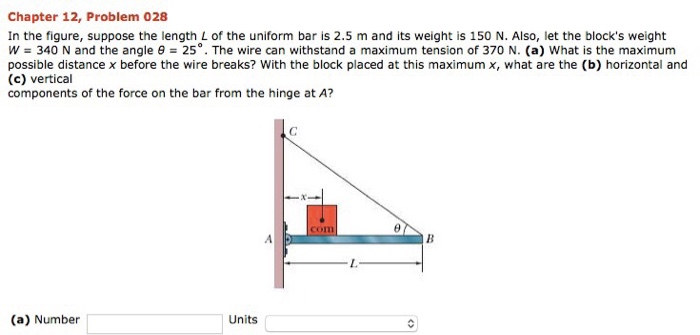Chapter 12, Problem 028 In the figure, suppose the length L of the uniform bar is 2.5 m and its weight is 150 N. Also, let the block's weight W = 340 N and the angle θ = 25°. The wire can withstand a maximum tension of 370 N. (a) what is the maximum possible distance x before the wire breaks? With the block placed at this maximum x, what are the (b) horizontal and (c) vertical components of the...

• ### In the figure, suppose the length L of the uniform bar is 2.9 m and its...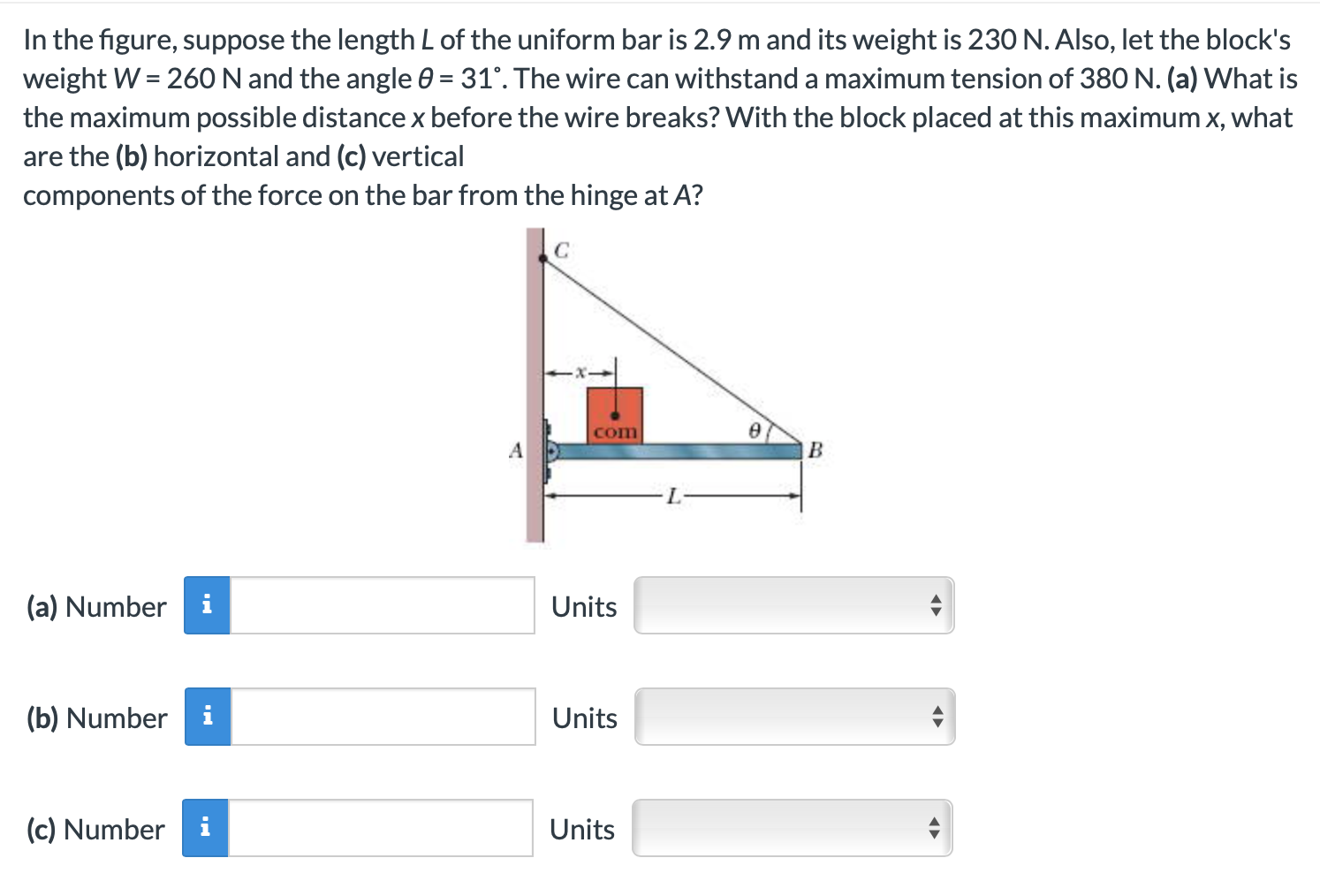In the figure, suppose the length L of the uniform bar is 2.9 m and its weight is 230 N. Also, let the block's weight W = 260 N and the angle 0 = 31°. The wire can withstand a maximum tension of 380 N. (a) What is the maximum possible distance x before the wire breaks? With the block placed at this maximum x, what are the (b) horizontal and (c) vertical components of the force on the bar...

Free Homework App